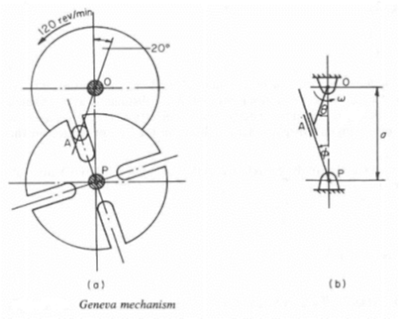# Engineering Mechanics-Statics

## Question No 1

Figure 1(a) shows a Geneva Mechanism whereby constant speed rotation of shaft O gives intermittent rotation of shaft P. The distance between shaft centers is 100 mm and the distance OA is 35 mm. For the position shown, draw proper space, velocity and acceleration diagrams for this mechanism, and find the angular velocity and acceleration of the slotted member.(a) Geneva mechanism  (b) A simplified model

## Solution

From Engineering Mechanics Dynamics (Ch 5), the provided figure it is clear that the triangle OPA will governs angular velocity and angular acceleration of this mechanism output. The first derivative of Ө and Ф will give the angular velocity and second derivative will give the angular acceleration of the OA and Slotted member respectively.

Using the law of sin to the make the equations and then taking derivative of them have given us the required angular velocity and acceleration of the slotted member. Reason for not using the PA side is that its length will change every instant; OA and OP side will remain constant and will give exact solution. Angular velocity and acceleration have same direction showing that the slotted member velocity will increase which is also clear from the provided figure.## Question No 2

Figure 2 shows a hatch cover that can pivot about a horizontal axis through O, under the control of a ram AP. The ram is supplied with hydraulic fluid so that it extends at a constant rate of 50 mm/s. Draw space, velocity and acceleration diagrams for the hatch just as it closes, that is, when θ = 0o, and hence find the angular acceleration of the hatch and of the arm at this instant.

Figure 2

## Solution

From Engineering Mechanics Dynamics (Ch 5), the motion of hatch and arm will be governed by the triangle AOP. See the free body diagram provided below, second derivative of angle α and β will give us the required angular acceleration of arm and hatch. Here rate of displacement of the arm AP is very important while calculating the angular acceleration of the arm and hatch so it must be added in the differential equations.

Assume Ө = 0 and calculate all the angles and length of sides using law of sin and then double derivate the equation to get the angular acceleration of the hatch and arm. Same sign on angular velocity and acceleration shows they have the same direction and arm and hatch is accelerating.

## Question No 3

Explain the significance of the equation in relation to the loading of the slender section illustrated by Figure 3. Using an appropriate graph explain the possible means of failure for a component in compression.

Figure 3

A suspension component has a 16mm outer diameter and a 12 mm inner diameter and is 0.4m in length. It is manufactured from steel with a Young’s modulus E=200GPa. Calculate a value for the critical compressive load before the onset of buckling.

The maximum compressive load that the pushrod will experience has been calculated as 2.5kN. Using the 2.5 kN as the critical load at which buckling occurs calculate a limiting internal diameter for the pushrod. Comment on the value calculated.

## Solution

According to Mechanics of Material (ch 10) no column is perfectly straight. At some stage compressive load result in the deformation of column. This failure of column under compression is called buckling and compression load at which it occurs is called critical compressive load. Critical compressive load can be calculated by the following formula

Pcr= K× n^2  ×  (π^2  ×E ×I)/L^2

In above equation two factors K and n are very important for our given problem. K and n are constants showing the restrains of two ends and buckling mode shape respectively. In our case slender have both ends pinned and have first mode of buckling, making K and n equal to 1. So we have the equation called Euler’s formula.

Pcr=  (π^2  ×E ×I)/L^2

Component under the compression load can undergo two types of failures; one is material failure and other the structure failure. Usually small components undergo the material failure. Ductile materials have plastics deformation before they fracture while ductile material fractures after the yield point under the compression load.

Structure like slender undergoes the structure failure called buckling due to the compressive forces. Higher value of L/r ratio for a slender can cause failure at lower stresses and vice versa.

As we are dealing with the pin ended slender and required to find the load at which the buckling start so both K and n are kept 1. At the critical compression load slender will start buckling, any load value below critical load cannot create buckling in slender.

When the critical compression load is taken 2.5KN thickness of the wall became very small about 0.13 mm. slender with that small thickness usually does not exist because the manufacturing that small thickness is very difficult.